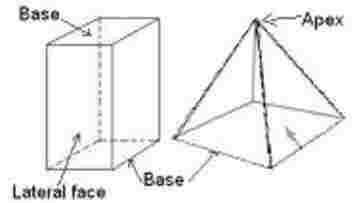Quadrangular Prism Area, Lateral Area and Volume Calculator

Calculate the area, lateral area and volume of a quadrangle prism.

Lateral Area:

 I want to calculate : Select Lateral Area Height Perimeter Units Select Unit meters centimeters feet inches
 Perimeter of base : Height : Lateral Area :

Total Area:

 I want to calculate : Select Total Area Lateral Area Area of base Units Select Unit meters centimeters feet inches
 Lateral Area : Area of base : Total area :

Volume :

 I want to calculate : Select Volume height Area of base Units Select Unit meters centimeters feet inches
 Area of the base : Height : Volume :Formula

We have following formulas in calculations:

Lateral Area = Perimeter_of_base x Height

Perimeter of base = Lateral_Area / Height

Hight = Lateral_Area / Perimeter_of_base

Total Area = Lateral_area + ( 2 x Area_of_the_base)

Lateral_area = Total Area - ( 2 x Area_of_the_base)

Area of the base = (Total Area - Lateral_area) / 2

Volume = Area_of_the base x Height

Height= Volume / Area_of_the base

Area_of_the base = Volume / Height